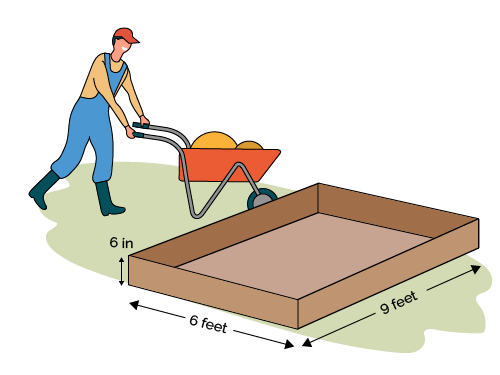# Square Feet to Cubic Yards Calculator

Created by Purnima Singh, PhD
Reviewed by Steven Wooding
Last updated: Jun 15, 2022

If you want to convert between square feet and cubic yards, our square feet to cubic yards calculator is the only tool you need. Just enter the area in square feet and the height/depth, and you will be able to calculate volume in cubic yards in no time.

To calculate volume in cubic yards from the base dimensions, i.e., length, width, and height, check out our cubic yard calculator.

Continue reading this article to know what a cubic yard is and how to convert square feet to cubic yards. You will also learn how to use our sq ft to cubic yards tool.

Before going any further, let us first try to understand what are square feet and cubic yards?

## Square feet and cubic yards

Square feet or $\rm ft^2$ is a unit of area, whereas, cubic yards or $\rm yd^3$ is a unit of volume.

Often we need to calculate the volume for construction or landscape material, for example, gravel, cement, soil, mulch, etc., and we use cubic yards to express this volume. For example, if you are preparing a flower bed and want to know how much mulch and soil you need.

Let us consider the given figure to understand the difference and relation between square feet and cubic yards. The figure shows a rectangular plot area that is 9 feet long and 6 feet wide. We can calculate the area of this rectangular plot as:\begin{align*} Area &= length \times width\\ & = 9\ \rm ft \times 6\ ft\\ & = 54\ \rm ft^2 \end {align*}

If we dig up the whole plot to 6-inch (or 0.5 ft) depth, the volume of the resulting bed would be:

\begin{align*} Volume &= Area \times depth\\ & = 54\ \rm ft^2 \times 0.5\ ft\\ & = 27\ \rm ft^3 \end {align*}

Using the relation $1\ \rm yd = 3\ ft$ or $1\ \rm yd^3 = 27\ ft^3$, we can write the above equation as:

\begin{align*} Volume &= 27\ \rm ft^3\\ & = 1\ \rm yd^3 \end {align*}

You can learn a similar conversion between volume and area in our gallons per square foot calculator, which is helpful if you're building a pool in your yard.

## How to convert square feet to cubic yards

Since square feet is a measure of area, and cubic yards is a measure of volume, to convert square feet to cubic yards, we need to follow the given steps:

1. Determine the area by using the appropriate formula and converting the unit of area to square feet.

2. Measure the depth/height of the area and convert it to feet as well.

3. Multiply the area and depth/height, and you will get volume in cubic feet.

4. Divide the volume in cubic feet by 27 to get the volume in cubic yards.

5. You can also use our square feet to cubic yards calculator for this purpose!

## How to use the square feet (sq. ft) to cubic yards calculator

Now let's see how we can use the square feet (sq. ft) to cubic yards calculator:

1. Enter the area of the surface in square feet. You can use our area calculator for this purpose.

2. Input the depth/height of the area. You can choose different units using the drop-down menu.

3. The sq. ft to cubic yards calculator will give you the volume in cubic yards.

4. You can also use this tool as cubic yards to square feet calculator.

## FAQ

### What is a cubic yard?

A cubic yard is a unit of volume. We can define a cubic yard as the volume of a cube with length, width, and height of one yard.

### How do I convert square feet to cubic yards?

To convert square feet to cubic yards, follow the given instructions:

1. Determine the length and breadth of the region of interest and calculate its area in sq. feet.

2. Find out the region's depth or height in feet.

3. Multiply results from steps 1 and 2 and divide the result by 27.

4. Congratulations! You have converted square feet to cubic yards.

### How do I convert cubic yards to square feet?

To convert cubic yards to square feet, proceed as follows:

1. Multiply the volume in cubic yards with 27 to get the volume in cubic feet.

2. Divide the volume by depth or height measured in feet.

3. You have calculated the area in square feet.

### How many cubic feet are in a cubic yard?

27 feet. One yard is equal to 3 feet. Hence to determine the number of cubic feet in a cubic yard, take the cube of both sides, and you will get 1 cubic yard = 27 cubic feet.

Purnima Singh, PhD
Area
ft²
Height/depth
in
Volume
cu yd
People also viewed…

### Car heat

The hot car calculator shows you how fast the interior of a car heats up during a summers day.

### Circumference

Use this free circumference calculator to find the area, circumference and diameter of a circle.

### Siding

This siding calculator finds the square footage and cost of siding for your house.

### Wallpaper

Wallpaper calculator finds the number of wallpaper rolls you need to buy for your room.